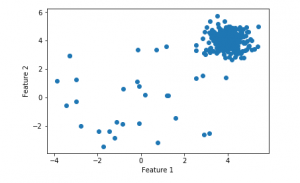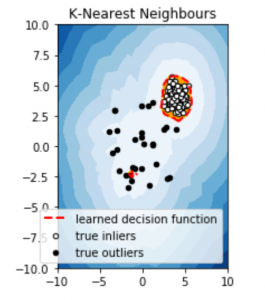# Machine Learning for Anomaly Detection

• Last Updated : 18 Aug, 2021

Anomaly Detection is the technique of identifying rare events or observations which can raise suspicions by being statistically different from the rest of the observations. Such “anomalous” behaviour typically translates to some kind of a problem like a credit card fraud, failing machine in a server, a cyber attack, etc.
An anomaly can be broadly categorized into three categories –

1. Point Anomaly: A tuple in a dataset is said to be a Point Anomaly if it is far off from the rest of the data.
2. Contextual Anomaly: An observation is a Contextual Anomaly if it is an anomaly because of the context of the observation.
3. Collective Anomaly: A set of data instances help in finding an anomaly.

Anomaly detection can be done using the concepts of Machine Learning. It can be done in the following ways –

1. Supervised Anomaly Detection: This method requires a labeled dataset containing both normal and anomalous samples to construct a predictive model to classify future data points. The most commonly used algorithms for this purpose are supervised Neural Networks, Support Vector Machine learning, K-Nearest Neighbors Classifier, etc.
2. Unsupervised Anomaly Detection: This method does require any training data and instead assumes two things about the data ie Only a small percentage of data is anomalous and Any anomaly is statistically different from the normal samples. Based on the above assumptions, the data is then clustered using a similarity measure and the data points which are far off from the cluster are considered to be anomalies.

We now demonstrate the process of anomaly detection on a synthetic dataset using the K-Nearest Neighbors algorithm which is included in the pyod module.
Step 1: Importing the required libraries

## Python3

 `import` `numpy as np` `from` `scipy ``import` `stats` `import` `matplotlib.pyplot as plt` `import` `matplotlib.font_manager` `from` `pyod.models.knn ``import` `KNN ` `from` `pyod.utils.data ``import` `generate_data, get_outliers_inliers`

Step 2: Creating the synthetic data

## Python3

 `# generating a random dataset with two features` `X_train, y_train ``=` `generate_data(n_train ``=` `300``, train_only ``=` `True``,` `                                                   ``n_features ``=` `2``)`   `# Setting the percentage of outliers` `outlier_fraction ``=` `0.1`   `# Storing the outliers and inliners in different numpy arrays` `X_outliers, X_inliers ``=` `get_outliers_inliers(X_train, y_train)` `n_inliers ``=` `len``(X_inliers)` `n_outliers ``=` `len``(X_outliers)`   `# Separating the two features` `f1 ``=` `X_train[:, [``0``]].reshape(``-``1``, ``1``)` `f2 ``=` `X_train[:, [``1``]].reshape(``-``1``, ``1``)`

Step 3: Visualising the data

## Python3

 `# Visualising the dataset` `# create a meshgrid` `xx, yy ``=` `np.meshgrid(np.linspace(``-``10``, ``10``, ``200``),` `                     ``np.linspace(``-``10``, ``10``, ``200``))`   `# scatter plot` `plt.scatter(f1, f2)` `plt.xlabel(``'Feature 1'``)` `plt.ylabel(``'Feature 2'``)`Step 4: Training and evaluating the model

## Python3

 `# Training the classifier` `clf ``=` `KNN(contamination ``=` `outlier_fraction)` `clf.fit(X_train, y_train)`   `# You can print this to see all the prediction scores` `scores_pred ``=` `clf.decision_function(X_train)``*``-``1`   `y_pred ``=` `clf.predict(X_train)` `n_errors ``=` `(y_pred !``=` `y_train).``sum``()` `# Counting the number of errors`   `print``(``'The number of prediction errors are '` `+` `str``(n_errors))`Step 5: Visualising the predictions

## Python3

 `# threshold value to consider a` `# datapoint inlier or outlier` `threshold ``=` `stats.scoreatpercentile(scores_pred, ``100` `*` `outlier_fraction)`   `# decision function calculates the raw ` `# anomaly score for every point` `Z ``=` `clf.decision_function(np.c_[xx.ravel(), yy.ravel()]) ``*` `-``1` `Z ``=` `Z.reshape(xx.shape)`   `# fill blue colormap from minimum anomaly` `# score to threshold value` `subplot ``=` `plt.subplot(``1``, ``2``, ``1``)` `subplot.contourf(xx, yy, Z, levels ``=` `np.linspace(Z.``min``(), ` `                  ``threshold, ``10``), cmap ``=` `plt.cm.Blues_r)`   `# draw red contour line where anomaly ` `# score is equal to threshold` `a ``=` `subplot.contour(xx, yy, Z, levels ``=``[threshold],` `                     ``linewidths ``=` `2``, colors ``=``'red'``)`   `# fill orange contour lines where range of anomaly` `# score is from threshold to maximum anomaly score` `subplot.contourf(xx, yy, Z, levels ``=``[threshold, Z.``max``()], colors ``=``'orange'``)`   `# scatter plot of inliers with white dots` `b ``=` `subplot.scatter(X_train[:``-``n_outliers, ``0``], X_train[:``-``n_outliers, ``1``],` `                                    ``c ``=``'white'``, s ``=` `20``, edgecolor ``=``'k'``) `   `# scatter plot of outliers with black dots` `c ``=` `subplot.scatter(X_train[``-``n_outliers:, ``0``], X_train[``-``n_outliers:, ``1``], ` `                                    ``c ``=``'black'``, s ``=` `20``, edgecolor ``=``'k'``)` `subplot.axis(``'tight'``)`   `subplot.legend(` `    ``[a.collections[``0``], b, c],` `    ``[``'learned decision function'``, ``'true inliers'``, ``'true outliers'``],` `    ``prop ``=` `matplotlib.font_manager.FontProperties(size ``=` `10``),` `    ``loc ``=``'lower right'``)`   `subplot.set_title(``'K-Nearest Neighbours'``)` `subplot.set_xlim((``-``10``, ``10``))` `subplot.set_ylim((``-``10``, ``10``))` `plt.show() `My Personal Notes arrow_drop_up
Recommended Articles
Page :